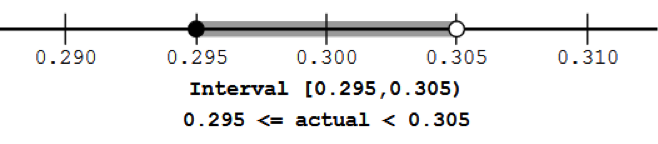$$\newcommand{\id}{\mathrm{id}}$$ $$\newcommand{\Span}{\mathrm{span}}$$ $$\newcommand{\kernel}{\mathrm{null}\,}$$ $$\newcommand{\range}{\mathrm{range}\,}$$ $$\newcommand{\RealPart}{\mathrm{Re}}$$ $$\newcommand{\ImaginaryPart}{\mathrm{Im}}$$ $$\newcommand{\Argument}{\mathrm{Arg}}$$ $$\newcommand{\norm}{\| #1 \|}$$ $$\newcommand{\inner}{\langle #1, #2 \rangle}$$ $$\newcommand{\Span}{\mathrm{span}}$$

# 23.8: C1.08: Interval Part 4

$$\newcommand{\vecs}{\overset { \rightharpoonup} {\mathbf{#1}} }$$ $$\newcommand{\vecd}{\overset{-\!-\!\rightharpoonup}{\vphantom{a}\smash {#1}}}$$$$\newcommand{\id}{\mathrm{id}}$$ $$\newcommand{\Span}{\mathrm{span}}$$ $$\newcommand{\kernel}{\mathrm{null}\,}$$ $$\newcommand{\range}{\mathrm{range}\,}$$ $$\newcommand{\RealPart}{\mathrm{Re}}$$ $$\newcommand{\ImaginaryPart}{\mathrm{Im}}$$ $$\newcommand{\Argument}{\mathrm{Arg}}$$ $$\newcommand{\norm}{\| #1 \|}$$ $$\newcommand{\inner}{\langle #1, #2 \rangle}$$ $$\newcommand{\Span}{\mathrm{span}}$$ $$\newcommand{\id}{\mathrm{id}}$$ $$\newcommand{\Span}{\mathrm{span}}$$ $$\newcommand{\kernel}{\mathrm{null}\,}$$ $$\newcommand{\range}{\mathrm{range}\,}$$ $$\newcommand{\RealPart}{\mathrm{Re}}$$ $$\newcommand{\ImaginaryPart}{\mathrm{Im}}$$ $$\newcommand{\Argument}{\mathrm{Arg}}$$ $$\newcommand{\norm}{\| #1 \|}$$ $$\newcommand{\inner}{\langle #1, #2 \rangle}$$ $$\newcommand{\Span}{\mathrm{span}}$$

Another method of reporting it would be$52700_{+50}^{-50}$ feet, which is usually only used if the distances on the two sides are not equal.

Example 10:     If a measured number is reported as 0.30 meters, what is the rounding precision, underline the significant digits, and find the interval of possible actual values.

Solution: The rounding precision is 0.01, which is one-hundredth. The significant digits are underlined: 0.30 The next-smaller rounded number is 0.29 and the next-larger rounded number is 0.31. We’ll write those as 0.290 and 0.300 and 0.310. (All numbers here are in meters.)This might also be reported as$0.30\pm0.005$ meters

Another method of reporting it would be$0.30_{+0.005}^{-0.005}$ meters, which is usually only used if the distances on the two sides are not equal.

Example 11:   If a measured number is reported as 12 meters,

1. what is the interval of actual values consistent with that,
2. what is the maximum amount that the actual value could differ from the reported value
3. what is the maximum amount of error as a percentage of the reported number?

Solution:

1. The interval of actual values is between 11.5 and 12.5 meters.
2. The maximum amount that the actual value could differ from the reported value is 0.5 meters.
3. To find the percentage of the maximum error as a percentage of the reported value, we must write it as a fraction, and then do the division and get a decimal fraction. Then convert that decimal to a percentage.$\frac{0.5\text{m}}{12\text{m}}=0.041666667$, which is 4.16666667%.

So the maximum error is about 4% of the reported value. This is called the relative error.

Example 12: If a measured number is reported as 12.0 meters,

1. what is the interval of actual values consistent with that,
2. what is the maximum amount that the actual value could differ from the reported value
3. what is the maximum amount of error as a percentage of the reported number?

Solution:

1. The interval of actual values is between 11.95 and 12.05 meters.
2. The maximum amount that the actual value could differ from the reported value is 0.05 meters.
3. To find the percentage of the maximum error as a percentage of the reported value, we must write it as a fraction, and then do the division and get a decimal fraction. Then convert that decimal to a percentage.$\frac{0.05\text{m}}{12\text{m}}=0.0041666667$, which is 0.416666667%.

So the maximum error is about 0.4% of the reported value. This relative error is one-tenth of the relative error in Example 11, reflecting the increased precision of the reported number.